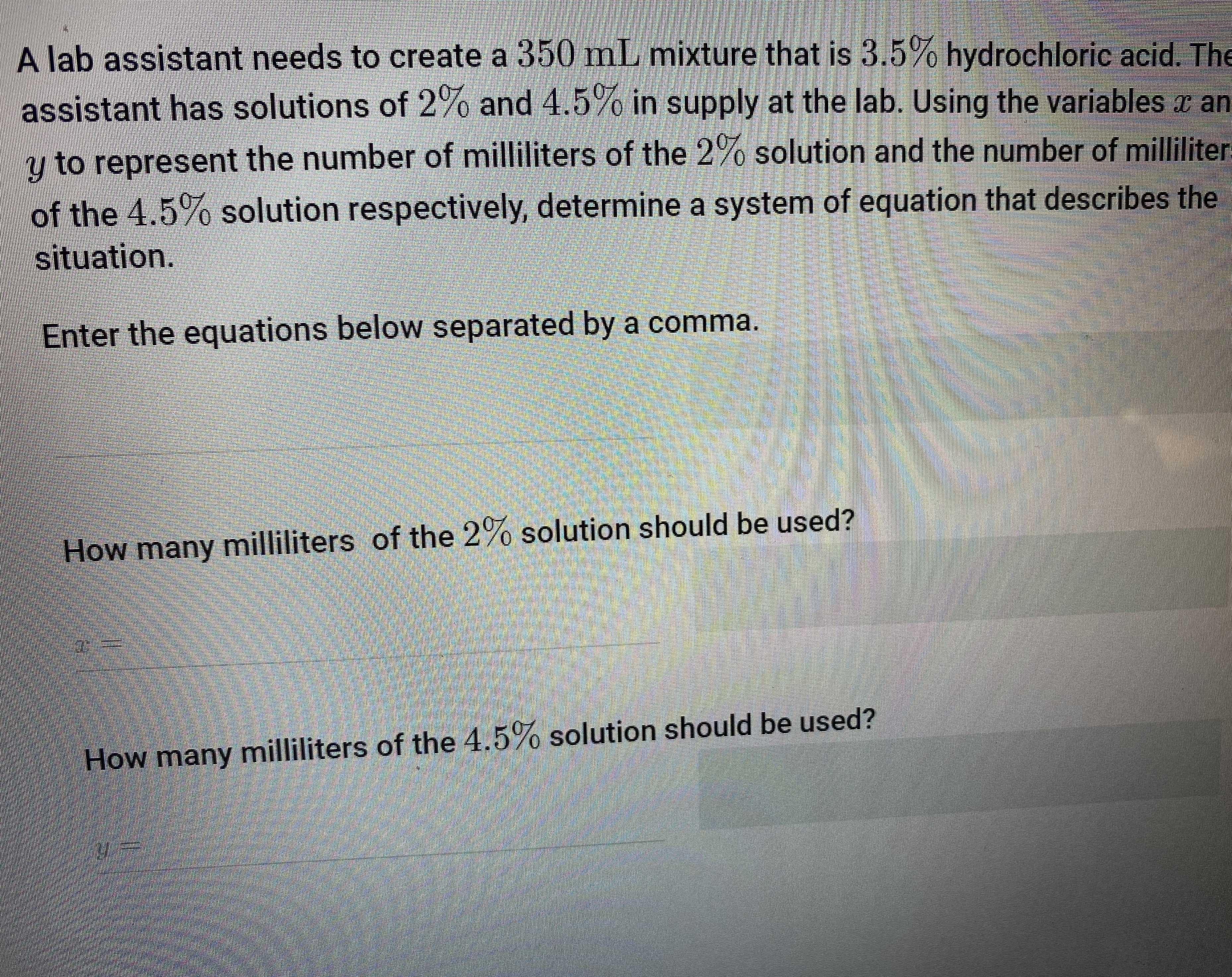### Still have math questions?

Algebra
QuestionA lab assistant needs to create a $$350 mL$$ mixture that is $$3.5 \%$$ hydrochloric acid. The assistant has solutions of $$2 \%$$ and $$4.5 \%$$ in supply at the lab. Using the variables $$x$$ an $$y$$ to represent the number of milliliters of the $$2 \%$$ solution and the number of milliliter of the $$4.5 \%$$ solution respectively, determine a system of equation that describes the situation.

Enter the equations below separated by a comma.

How many milliliters of the $$2 \%$$ solution should be used?

x=

How many milliliters of the $$4.5 \%$$ solution should be used?

$$y =$$